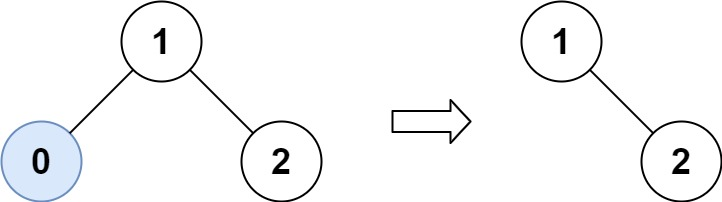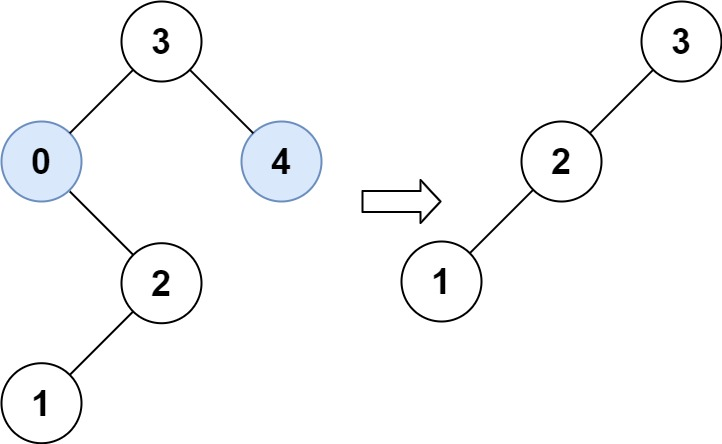## 669. Trim a Binary Search Tree

Given the `root` of a binary search tree and the lowest and highest boundaries as `low` and `high`, trim the tree so that all its elements lies in `[low, high]`. Trimming the tree should not change the relative structure of the elements that will remain in the tree (i.e., any node's descendant should remain a descendant). It can be proven that there is a unique answer.

Return the root of the trimmed binary search tree. Note that the root may change depending on the given bounds.

Example 1:```Input: root = [1,0,2], low = 1, high = 2
Output: [1,null,2]
```

Example 2:```Input: root = [3,0,4,null,2,null,null,1], low = 1, high = 3
Output: [3,2,null,1]
```

Example 3:

```Input: root = , low = 1, high = 2
Output: 
```

Example 4:

```Input: root = [1,null,2], low = 1, high = 3
Output: [1,null,2]
```

Example 5:

```Input: root = [1,null,2], low = 2, high = 4
Output: 
```

Constraints:

• The number of nodes in the tree in the range `[1, 104]`.
• `0 <= Node.val <= 104`
• The value of each node in the tree is unique.
• `root` is guaranteed to be a valid binary search tree.
• `0 <= low <= high <= 104`

## Rust Solution

``````struct Solution;
use rustgym_util::*;

impl Solution {
if let Some(node) = root.clone() {
let mut node = node.borrow_mut();
let left = node.left.take();
let right = node.right.take();
if node.val > r {
return Self::trim_bst(left, l, r);
}
if node.val < l {
return Self::trim_bst(right, l, r);
}
node.left = Self::trim_bst(left, l, r);
node.right = Self::trim_bst(right, l, r);
root
} else {
None
}
}
}

#[test]
fn test() {
let root = tree!(1, tree!(0), tree!(2));
let res = tree!(1, None, tree!(2));
assert_eq!(Solution::trim_bst(root, 1, 2), res);
let root = tree!(3, tree!(0, None, tree!(2, tree!(1), None)), tree!(4));
let res = tree!(3, tree!(2, tree!(1), None), None);
assert_eq!(Solution::trim_bst(root, 1, 3), res);
}
``````

Having problems with this solution? Click here to submit an issue on github.